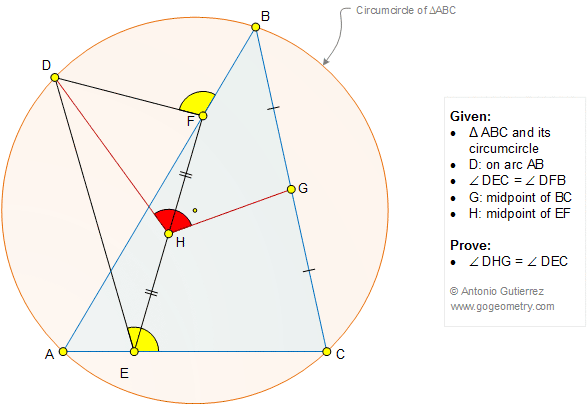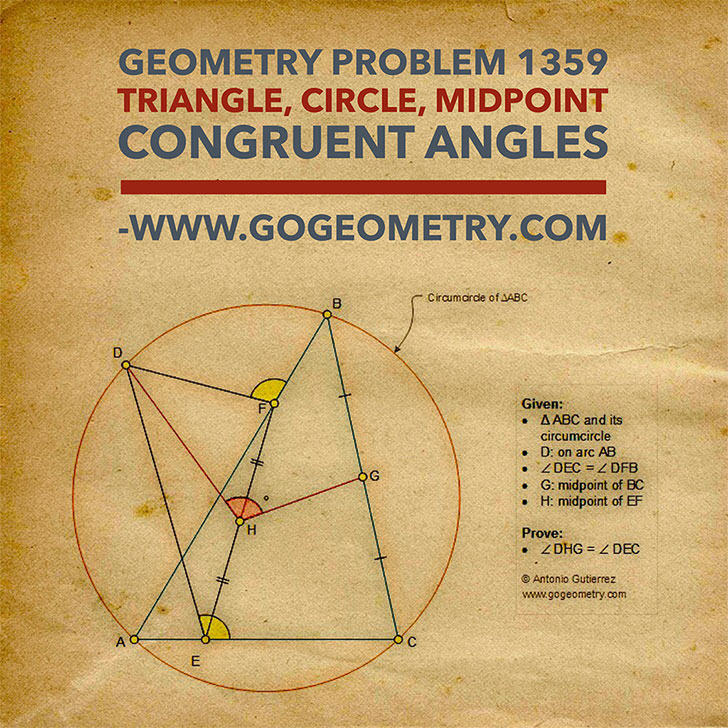# Geometry Problem 1359: Triangle, Circumcircle, Congruent Angles, Midpoints.

### Proposition

A triangle ABC is inscribed in a circle and a point D is on the arc AB. Angles DEC and DFB are congruent, G is the midpoint of BC and H is the midpoint of EF. (See the figure below). Prove that angles DHG and DEC are congruent### Sketch of problem 1359